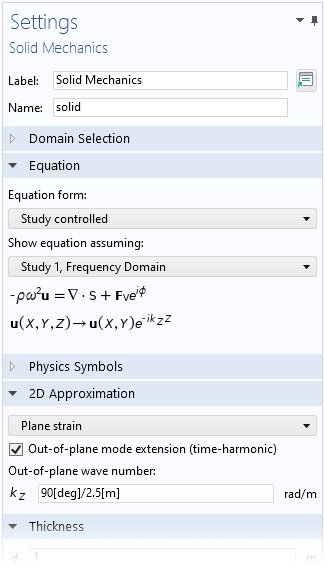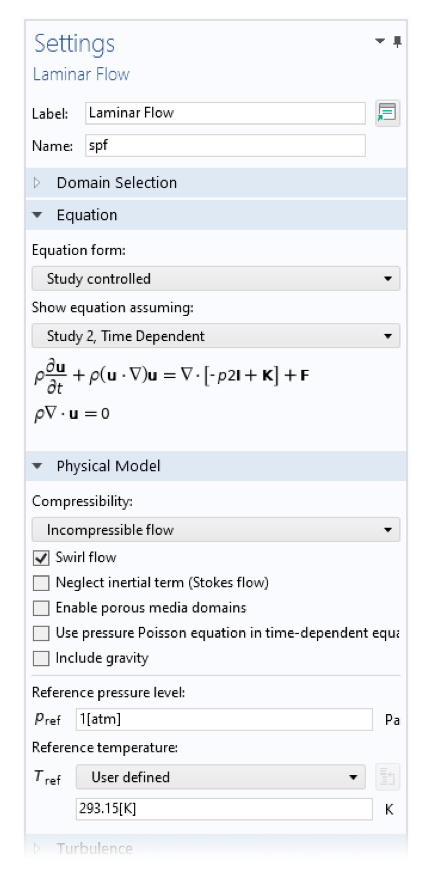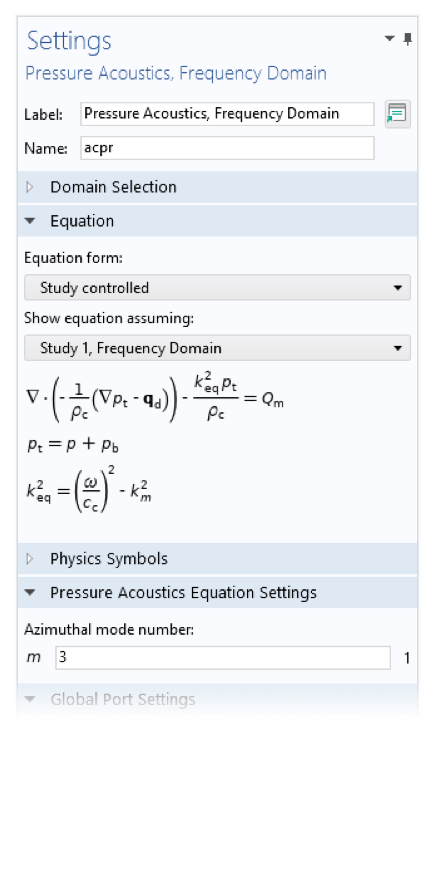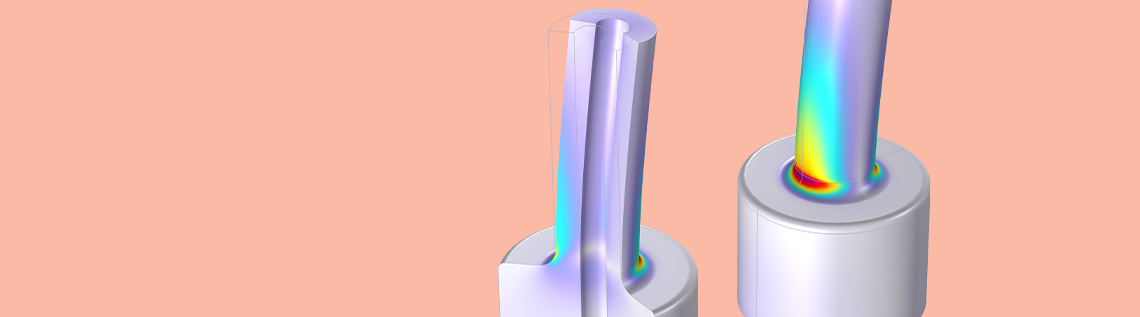# 通过二维轴对称建模分析扭转问题

2022年 2月 8日

### 位移梯度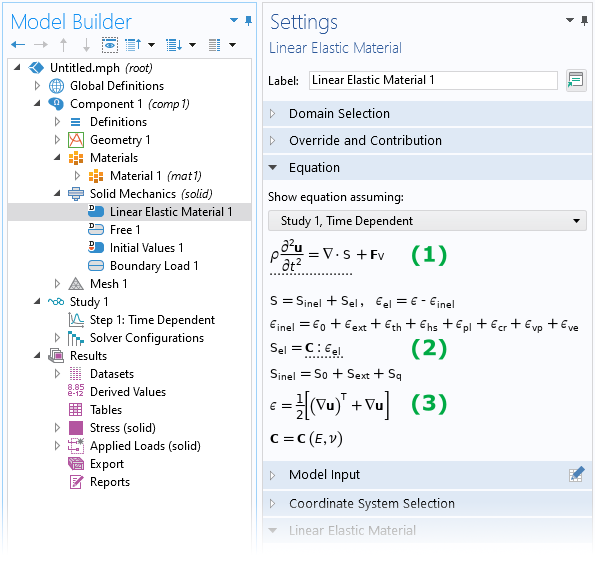\mathbf{x}+ \mathrm{d}\mathbf{x} = \mathbf{X} + \mathrm{d}\mathbf{X} + \mathbf{u}(\mathbf{X}+\mathrm{d}\mathbf{X}, t)

\mathbf{x}
，是点 \textrm{P}_1\textrm{P}_2 在变形配置中的一小步。

\mathrm{d}\mathbf{x} &= \mathbf{X} + \mathrm{d}\mathbf{X} + \mathbf{u}(\mathbf{X}+\mathrm{d}\mathbf{X}, t) – \mathbf{x} \\[1mm]
&= \mathbf{X} + \mathrm{d}\mathbf{X} + \mathbf{u}(\mathbf{X}+\mathrm{d}\mathbf{X}, t) – \left[ \mathbf{X} + \mathbf{u}(\mathbf{X},t) \right] \\[1mm]
&= \mathrm{d}\mathbf{X} + \mathbf{u}(\mathbf{X}+\mathrm{d}\mathbf{X}, t) – \mathbf{u}(\mathbf{X},t)
= \mathrm{d}\mathbf{X} + \mathrm{d}\mathbf{u} \\[1mm]
& = \mathrm{d}\mathbf{X} + \left( \nabla \mathbf{u} \right) \mathrm{d} \mathbf{X} \\[1mm]
& = \left( I + \nabla \mathbf{u} \right) \mathrm{d}\mathbf{X}

\nabla \mathbf{u} =
\left[
{\begin{array}{ccc}
\frac{\partial u}{\partial X} & \frac{\partial u}{\partial Y} & \frac{\partial u}{\partial Z} \\
\frac{\partial v}{\partial X} & \frac{\partial v}{\partial Y} & \frac{\partial v}{\partial Z} \\
\frac{\partial w}{\partial X} & \frac{\partial w}{\partial Y} & \frac{\partial w}{\partial Z} \\
\end{array} }
\right]

\nabla \mathbf{u} =
\left[
{\begin{array}{ccc}
\frac{\partial u}{\partial R} & \frac{1}{R} \frac{\partial u}{\partial \Phi}-\frac{v}{R} & \frac{\partial u}{\partial Z} \\
\frac{\partial v}{\partial R} & \frac{1}{R} \frac{\partial v}{\partial \Phi}+\frac{u}{R} & \frac{\partial v}{\partial Z} \\
\frac{\partial w}{\partial R} & \frac{1}{R} \frac{\partial w}{\partial \Phi} & \frac{\partial w}{\partial Z} \\
\end{array} }
\right]

### 添加扭转…

1. 添加周向位移分量作为一个新的因变量
2. 显示新的用户输入，例如在圆周方向上施加载荷、弹簧或阻尼
3. 修改位移梯度的定义

\nabla \mathbf{u} &=
\left[
{\begin{array}{ccc}
\frac{\partial u}{\partial R} & -\frac{v}{R} & \frac{\partial u}{\partial Z} \\
\frac{\partial v}{\partial R} & \frac{u}{R} & \frac{\partial v}{\partial Z} \\
\frac{\partial w}{\partial R} & 0 & \frac{\partial w}{\partial Z} \\
\end{array} }
\right]

### 圆周模式扩展

\mathbf{\hat{u}} = \mathbf{u} (R,Z) \, e^{-im\Phi} = \mathbf{u}
(R,Z) \left[ \cos (m \Phi) – i \, \sin (m \Phi) \right]

\nabla\mathbf{\hat{u}} =
\begin{bmatrix}
\frac{\partial u}{\partial R}&-\frac{v}{R}& \frac{\partial u}{\partial Z} \\
\frac{\partial v}{\partial R} &\frac{u}{R}& \frac{\partial v}{\partial Z} \\
\frac{\partial w}{\partial R}&0& \frac{\partial w}{\partial Z} \end{bmatrix} – i \frac{m}{R} \begin{bmatrix}
0 & u & 0 \\
0 & v & 0 \\
0 & w & 0
\end{bmatrix}

1. m=0，对应于一个常数移位
2. m=1，可以描述二维轴对称中的弯曲变形

 m=0m=1m=2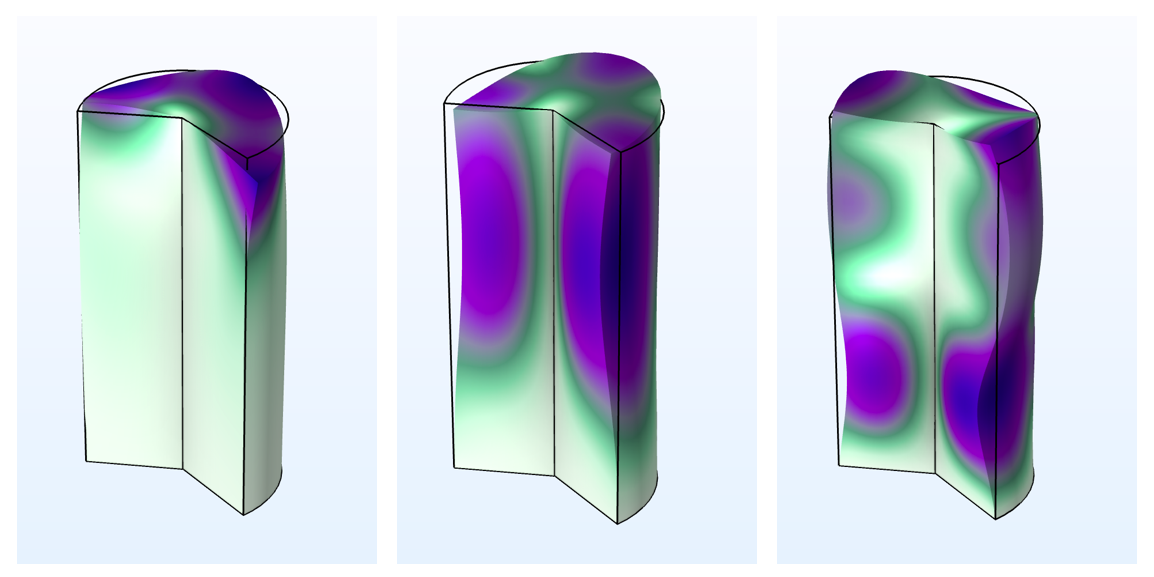{Hz}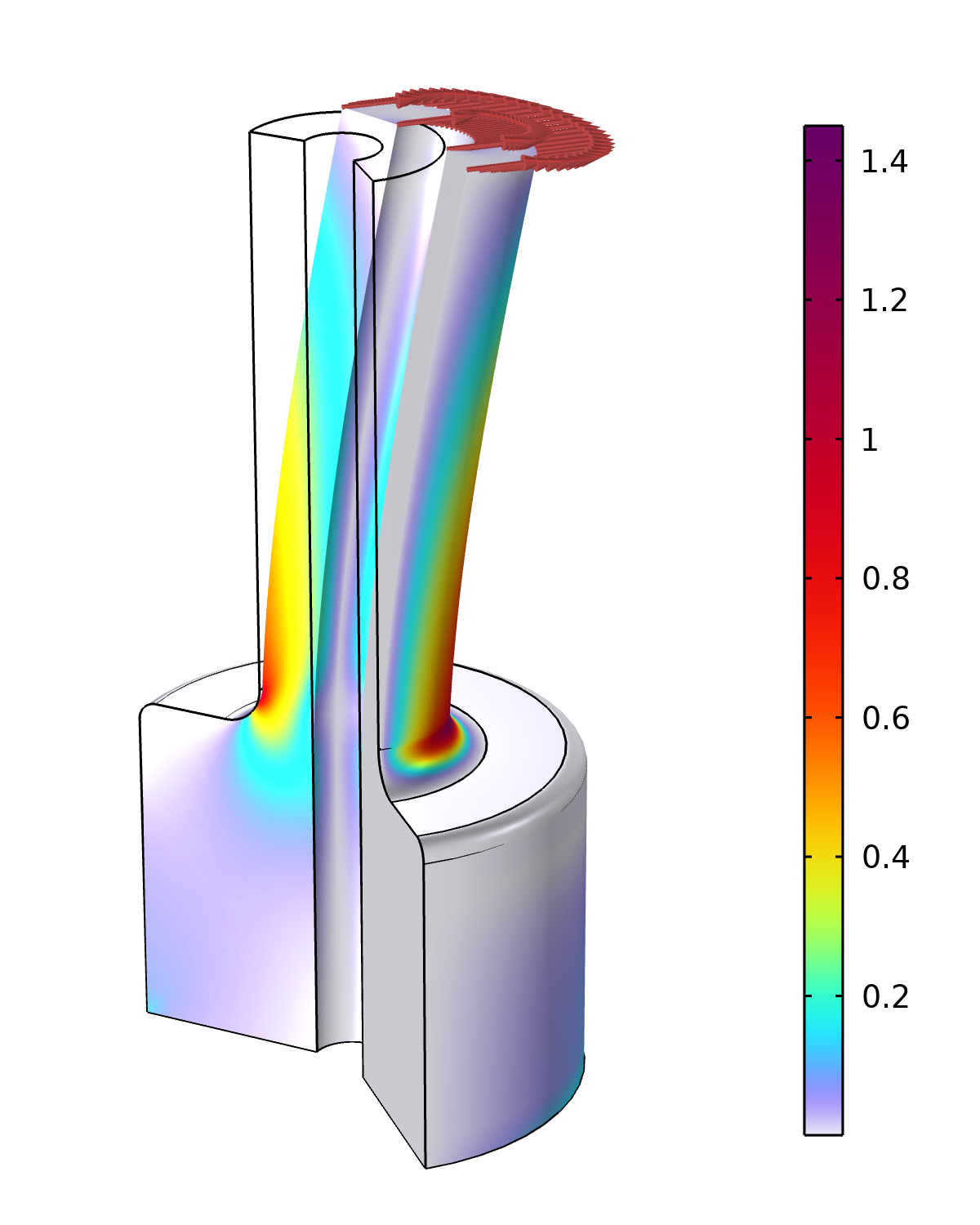### 其他结构力学接口呢？